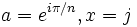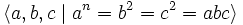# Dicyclic group

WARNING: POTENTIAL TERMINOLOGICAL CONFUSION: Please don't confuse this with metacyclic group
WARNING: POTENTIAL TERMINOLOGICAL CONFUSION: Please don't confuse this with dihedral group
This article defines a group property: a property that can be evaluated to true/false for any given group, invariant under isomorphism
View a complete list of group properties
VIEW RELATED: Group property implications | Group property non-implications |Group metaproperty satisfactions | Group metaproperty dissatisfactions | Group property satisfactions | Group property dissatisfactions

This is a family of groups parametrized by the natural numbers, viz, for each natural number, there is a unique group (upto isomorphism) in the family corresponding to the natural number. The natural number is termed the parameter for the group family

## Definition

The dicyclic group, also called the binary dihedral group with parameter$n$ is defined in the following equivalent ways:$\langle a,x \mid a^{2n}=e, x^2 = a^n, xax^{-1} = a^{-1} \rangle$

Here,$e$ is the identity element.

• It has the following faithful representation as a subgroup of the quaternions:$a = e^{i\pi/n}, x = j$.
• It is the binary von Dyck group with parameters$(n,2,2)$, i.e., it has the presentation:$\langle a,b,c \mid a^n = b^2 = c^2 = abc \rangle$.

The dicyclic group with parameter$n$ has order$4n$, and it is an extension of a cyclic group of order$2n$ by a cyclic group of order 2.

### Equivalence of definitions

Further information: equivalence of presentations of dicyclic group

## Cases

The dicyclic group has some alternate descriptions in specific cases.

Case on$n$ Examples Description of dicyclic group
Odd number$n = 3$, so dicyclic group:Dic12 Semidirect product of cyclic normal subgroup of order$n$ (generated by$a^2$) and group of order 4 generated by$x$ (in the first presentation) or$b$ (in the second). The latter element conjugates$a$ to its inverse.

## Arithmetic functions

Here, the$n$ is as in the parametrization. The order of the group is$4n$.

Function Value Explanation
order$4n$
exponent least common multiple of$4$ and$2n$
nilpotency class$k + 1$ if$n = 2^k$, undefined otherwise.
derived length 2 for$n \ge 2$
number of conjugacy classes$n + 3$
number of subgroups$\sigma(n) + d(2n)$ where$\sigma$ is the divisor sum function and$d$ is the divisor count function

## Group properties

Property Satisfied Explanation
Abelian group No for$n \ge 2$.
Nilpotent group Yes only for$n$ a power of two.
Solvable group Yes
Supersolvable group Yes
Metacyclic group Yes
Ambivalent group Yes for$n$ even, no for$n$ odd
Rational group Yes only for$n =2$, i.e., the quaternion group

## Particular cases

### For small values

Note that all dicyclic groups are metacyclic and hence supersolvable. A dicyclic group is nilpotent if and only if it is of order$2^k$ for some$k$. It is abelian only if it has order 4.

Order of group Degree Common name for the group Comment
4 1 Cyclic group:Z4 Not typically considered a dicyclic group
8 2 Quaternion group
12 3 Dicyclic group:Dic12
16 4 Generalized quaternion group:Q16

## Elements

Further information: Element structure of dicyclic groups

The dicyclic group of order$4n$ has$n+3$ conjugacy classes. In the discussion below, we use the presentation:$\langle a,x \mid a^{2n}=e, x^2 = a^n, x^{-1}ax = a^{-1} \rangle$

The elements are:

1. The identity element. (1)
2. The unique central non-identity element, which is given by$a^n = x^2$. (1)
3. The remaining elements in$\langle a \rangle$. There are$2n - 2$ of these elements, and they occur in conjugacy classes of size two: each element is conjugate to its inverse. There are thus$n - 1$ conjugacy classes of size$2$ each.
4. The elements outside$\langle a \rangle$ come in two conjugacy classes: the conjugacy class of$x$, which contains all elements of the form$a^{2k}x$, and the conjugacy class of$ax$. These two conjugacy classes are related by an outer automorphism and each has$n$ elements.